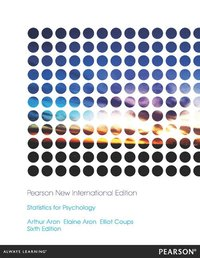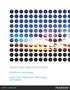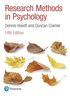Fler böcker inom
Format
Häftad (Paperback)
Språk
Engelska
Antal sidor
728
Utgivningsdatum
2013-11-01
Upplaga
6
Förlag
Pearson
Medarbetare
Aron, Elaine N. / Coups, Elliot J. / Publishing, Cole
Dimensioner
274 x 215 x 23 mm
Vikt
1550 g
Antal komponenter
1
ISBN
9781292040295

### Du kanske gillar# Statistics for Psychology: Pearson New International Edition

Häftad Engelska, 2013-11-01
759
Skickas inom 5-8 vardagar.
Gratis frakt inom Sverige över 159 kr för privatpersoner.
Emphasizing meaning and concepts, not just symbols and numbers

Statistics for Psychology, 6th edition places definitional formulas center stage to emphasize the logic behind statistics and discourage rote memorization. Each procedure is explained in a direct, concise language and both verbally and numerically.

MyStatLab is an integral part of the Statistics course. MyStatLab gives students practice with hundreds of homework problems. Every problem includes tools to help students understand and solve each problem - and grades all of the problems for instructors. MyStatLab also includes tests, quizzes, eText, a Gradebook, a customizable study plan, and much more.

Learning Goals

Upon completing this book, readers should be able to:

• Know both definitional and numerical formulas and how to apply them
• Understand the logic behind each formula
• Expose students to the latest thinking in statistical theory and application
• Prepare students to read research articles
• Learn how to use SPSS

## Passar bra ihop

1.2. +
3.De som köpt den här boken har ofta också köpt Research Methods in Psychology av Dennis Howitt (häftad).

## Kundrecensioner

Har du läst boken? Sätt ditt betyg »

## Fler böcker av Arthur Aron

• ### Handbook of Closeness and Intimacy

#### Debra J Mashek, Arthur Aron

This handbook brings together the latest thinking on the scientific study of closeness and intimacy from some of the most active and widely recognized relationship scholars in social and clinical psychology, communication studies, and related disc...

• ### Statistics for The Behavioral and Social Sciences: Pearson New International Edition

#### Arthur Aron

For one-quarter/semester courses that focus on the basics in statistics or combine statistics with research methods. &nbsp; The&nbsp;fifth edition of Statistics for the Behavioral and Social Sciences builds off an already well-established&nbsp;app...

## Innehållsförteckning

In this Section:

1. Brief Table of Contents

2. Full Table of Contents

1. BRIEF TABLE OF CONTENTS

Chapter 1   Displaying the order in a group of numbers

Chapter 2   Central tendency and variability

Chapter 3   Some key ingredients for inferential statistics: Z scores, the normal curve, sample versus

population, and probability

Chapter 4   Introduction to hypothesis testing

Chapter 5   Hypothesis testing with means of samples

Chapter 6   Making sense of statistical significance: Effect size and statistical power

Chapter 7   Introduction to the t test: Single sample and dependent means

Chapter 8   The t test for independent means

Chapter 9   Introduction to the analysis of variance

Chapter 10 Factorial analysis of variance

Chapter 11 Correlation

Chapter 12 Prediction

Chapter 13 Chi-square tests

Chapter 14 Strategies when population distributions are not normal: Data transformations and rank-order

tests

Chapter 15 Integration and the general linear model and Making sense of advanced statistical procedures in

research articles

2. FULL TABLE OF CONTENTS

Chapter 1: Displaying the order in a group of numbers

The Two Branches of Statistical Methods

Some Basic Concepts

Frequency Tables

Histograms

Shapes of Frequency Distributions

Controversy: Misleading Graphs

Frequency Tables and Histograms in Research Articles

Summary

Key Terms

Example Worked-Out Problems

Practice Problems

Using SPSS

Chapter Note

Chapter 2: Central tendency and variability

Central Tendency

Variability

Controversy: The Tyranny of the Mean

Central Tendency and Variability in Research Articles

Summary

Key Terms

Example Worked-Out Problems

Practice Problems

Using SPSS

Chapter Note

Chapter 3: Some key ingredients for inferential statistics: Z scores, the normal  curve, sample versus

population, and probability

Z Scores

The Normal Curve

Sample and Population

Probability

Controversies: Is the Normal Curve Really So Normal? And Using Nonrandom Samples

Z Scores, Normal Curves, Samples and Populations, and Probabilities in Research Articles

Advanced Topics: Probability Rules and Conditional Probabilities

Summary

Key Terms

Example Worked-Out Problems

Practice Problems

Using SPSS

Chapter Note

Chapter 4: Introduction to hypothesis testing

A Hypothesis-Testing Example

...